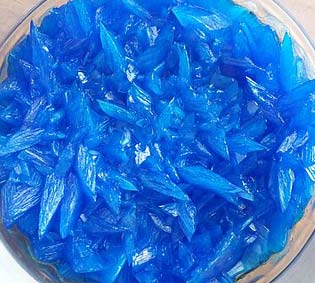Chemistry Edexcel GCSE:
Acids 1

Page 1

1. Rain water is weakly acidic because it contains dissolved carbon dioxide. Which of these is a possible pH for rain water?

2. Litmus, phenolphthalein and methyl orange are all indicators which can be used to distinguish between acids and alkalis.

What are the correct colours of these indicators in alkali?

 litmus phenolphthalein Methyl orange A blue colourless yellow B blue pink yellow C blue pink red D red colourless red
3. Which of the following statements about pH is correct?
• A.   The lower the concentration of hydroxide ions in a solution the higher the pH
• B.   The pH decreases when calcium oxide is added to dilute hydrochloric acid
• C.   The higher the concentration of hydrogen ions in a solution the lower the pH
• D.   Acidic solutions have a pH value above 7
4. Sulfuric acid is a strong acid. A strong acid is one which..
• A.   is fully dissociated into ions in aqueous solution
• B.   is partially dissociated into ions in aqueous solution
• C.   contains a large amount of acid dissolved in water
• D.   contains a small amount of acid dissolved in water
5. Which is NOT a typical characteristic of acids?
• A.   They react with metal oxides producing hydrogen
• B.   They react with carbonates producing carbon dioxide
• C.   They react with alkalis producing water
• D.   They turn blue litmus paper red

6. Acids form salts. Select the row that correctly states the type of salt formed from each acid.

 Hydrochloric acid Sulfuric acid Nitric acid A chlorate sulfide nitride B chlorate sulfate nitrate C chloride sulfate nitride D chloride sulfate nitrate

Q7-9:
A student prepared a sample of hydrated copper(II)sulfate crystals by reacting excess copper(II)carbonate with dilute sulfuric acid.7. The equation for this reaction with the correct state symbols is ..
• A.  CuCO3(s) + H2SO4(l)  CuSO4(l) + H2O(l) + CO2(g)
• B.  CuCO3(s) + H2SO4(aq)  CuSO4(aq) + H2O(l) + CO2(g)
• C.  CuCO3(s) + H2SO4(l)  CuSO4(aq) + H2O(l) + CO2(g)
• D.  CuCO3(aq) + H2SO4(aq)  CuSO4(aq) + H2O(l) + CO2(g)
8. How would the student know when she had added an excess of copper(II)carbonate?
• A.   the solution would turn blue in colour
• B.   the temperature would rise
• C.   effervescence would stop and solid would remain in the mixture
• D.   all the solid would disappear

9. What techniques would the student use to:

(a) separate the excess copper(II)carbonate from the mixture?
(b) obtain hydrated copper(II)sulfate crystals from the solution?

 Separate excess copper(II) carbonate Obtain hydrated copper(II)sulfate crystals A filtration crystallisation B evaporation distillation C filtration distillation D evaporation crystallisation

10. Which statements are NOT true about bases?

 I. Bases reacts with acids to form salt and water only II. Bases turn damp blue litmus paper to red III. An alkali is an insoluble base

• A.   I and II only
• B.   I and III only
• C.   II and III only
• D.   I, II and III